Vectors and conics

This free course is available to start right now. Review the full course description and key learning outcomes and create an account and enrol if you want a free statement of participation.

Free course

3 Dot product

3.1 Definition, properties and some applications

In the previous section we saw how to add two vectors and how to multiply a vector by a scalar, but we did not consider how to multiply two vectors. There are two different ways in which we can multiply two vectors, known as the dot product (or scalar product) and the vector product. They are given these names because the result of the first is a scalar and the result of the second is a vector. (We shall not consider vector products in this course.)

In the audio section we explain the definition of the dot product and investigate some of its basic properties. In addition, we see how the dot product can be used to find the angle between two vectors, to give a condition for two vectors to be orthogonal (that is, at right angles) and to find the projection of one vector onto another vector.

Listen to the audio below as you work through the following frames.

Click 'Play' to listen to Audio Clip 1 while viewing frames 1–7 in the main text below.

Interactive feature not available in single page view (see it in standard view).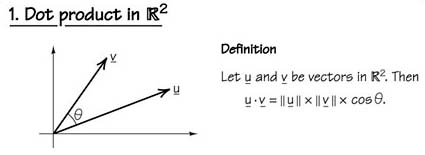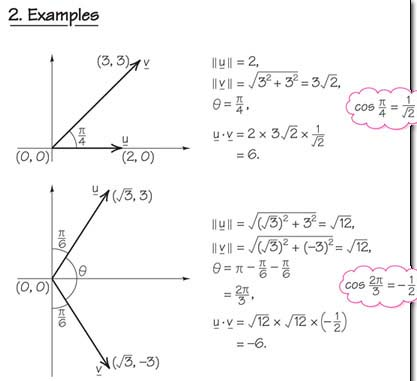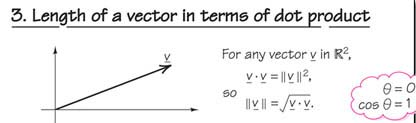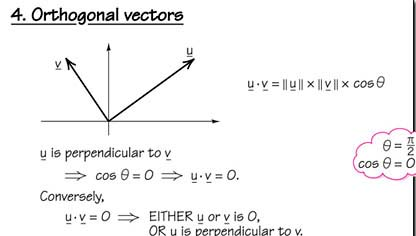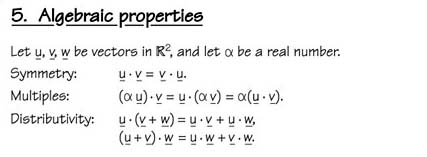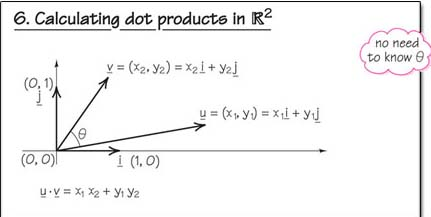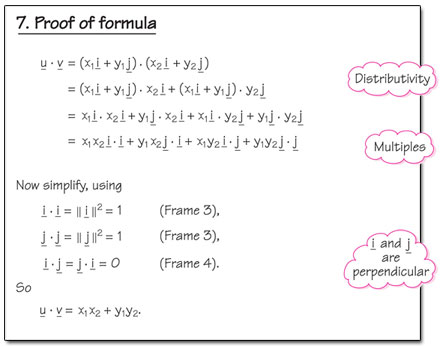Click 'Play' to listen to Audio Clip 2 while viewing frames 8–12 in the main text below.

Interactive feature not available in single page view (see it in standard view).

Example 37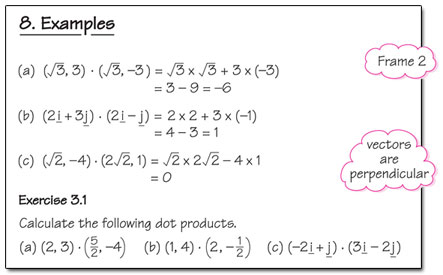We use the formula for the dot product given in Frame 6 Section 3..

• (a)

•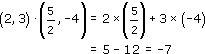• (b)

•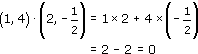• (c)

•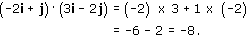Example 38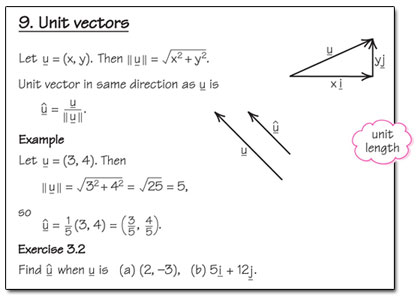• (a) When u = (2, −3), the length of u is

•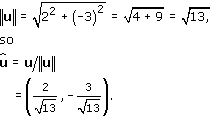• so

•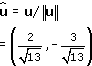• (b) When u = 5i + 12j, the length of u is

•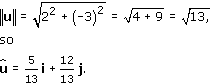• so

•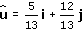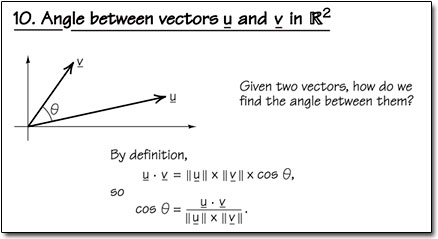Example 39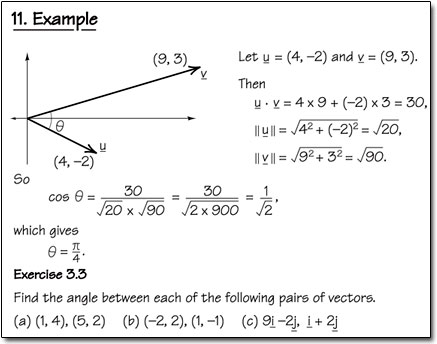In each case we use the formula for the angle between two vectors given in Frame 10 Section 3.1 , letting u denote the first vector of the pair, v the second vector and θ the angle between the two vectors.

• (a) Here u · v = (1, 4) · (5, 2) = 5 + 8 = 13,

•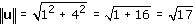• and

•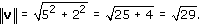• Then

•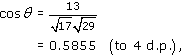• so

•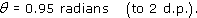• (b) Here u · v = (−2, 2) · (1, −1) = −2 −2 = −4,

•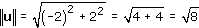• and

•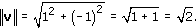• Then

•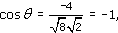• so

•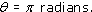• (c) Here

•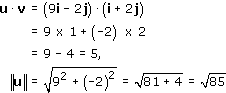• and

•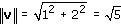• Then

•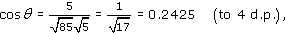• so

•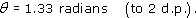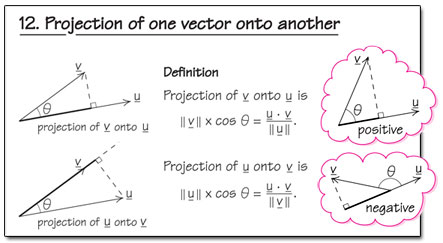Click 'Play' to listen to Audio Clip 3 while viewing frames 13–17 in the main text below.

Interactive feature not available in single page view (see it in standard view).

Example 40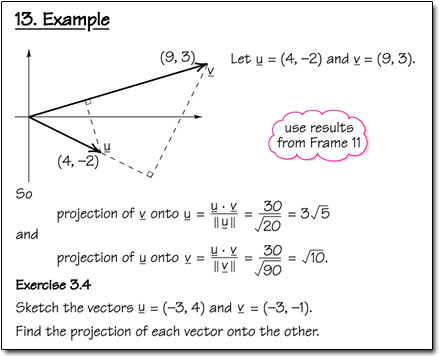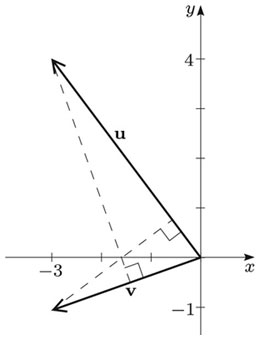To calculate the projections of u onto v and v onto u, we need the following: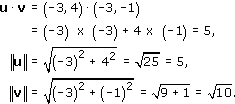Then the projection of v onto u is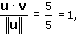and the projection of u onto v is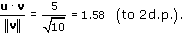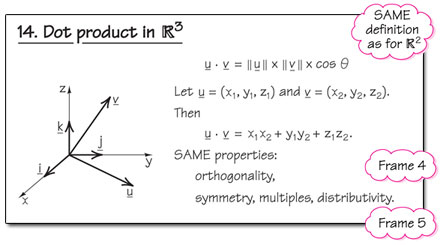Example 41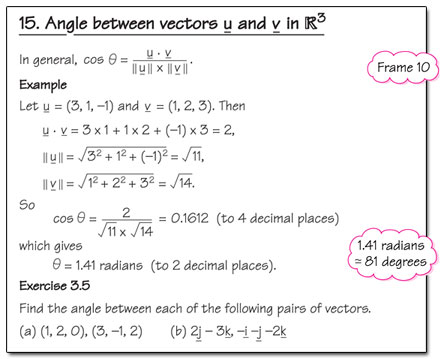In each case we use the formula for the angle between two vectors given in Frame 10, letting u denote the first vector of the pair, v the second vector and θ the angle between the two vectors.

• (a) Here u · v = (1, 2, 0) · (3, −1, 2) = 3 − 2 + 0 = 1,

•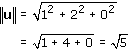• and

•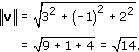• Then

•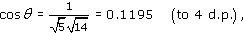• so

•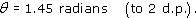• (b)Here

•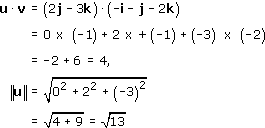• and

•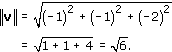• Then

•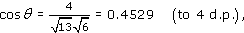• so

•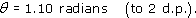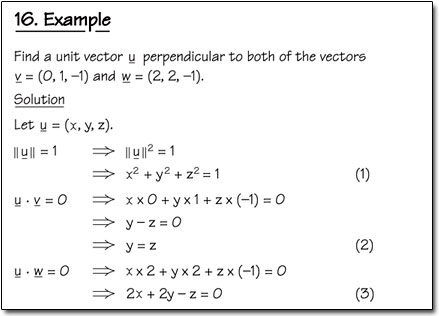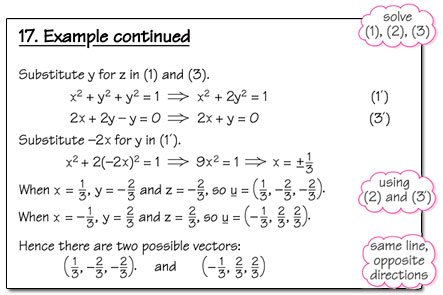M208_1